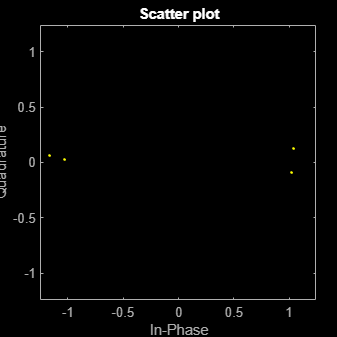# lteACKDecode

HARQ-ACK channel decoding

## Syntax

``[out,blkerr] = lteACKDecode(chs,in)``

## Description

example

````[out,blkerr] = lteACKDecode(chs,in)` performs block decoding on soft input data `in`, assumed to be encoded using the procedure defined for HARQ-ACK in Section 5.2.2.6 of , for PUSCH channel transmission configuration `chs`. The decoded output, `out`, is a vector of length `OACK`, the number of uncoded HARQ-ACK bits transmitted. NoteIf `NBundled` is 0, TDD ACK-NACK descrambling is disabled. Multiple codewords can be parameterized by two different forms of the `chs` structure. Each codeword can be defined by separate elements of a 1-by-2 structure array, or the codeword parameters can be combined together in the fields of a single scalar, or 1-by-1, structure. Any scalar field values apply to both codewords and a scalar `chs.NLayers` is the total number. See UL-SCH Parameterization for further details. The block decoding is performed separately on each soft input data codeword using a maximum likelihood (ML) approach, assuming that `in` has been demodulated and equalized to best restore the originally transmitted values. The HARQ-ACK decoder performs different types of block decoding depending upon the number of uncoded HARQ-ACK bits to be recovered (`OACK`). For `OACK` less than 3 bits, the decoder assumes the bits are encoded using the procedure defined in TS 36.212 , Section 5.2.2.6. For decoding between 3 and 11 HARQ-ACK bits, the decoder assumes the bits are block encoded using the procedure defined in TS 36.212 , Section 5.2.2.6.4. For decoding between 12 and 22 bits, the decoder performs the inverse procedure described in TS 36.212 , Section 5.2.2.6.5. For decoding more than 22 bits, the decoder performs rate-matching, tail-biting Viterbi decoding, and 8-bits CRC decoding, which is the inverse procedure described in Section 5.2.2.6. ```

## Examples

collapse all

Show the block decoding of 3 coded HARQ-ACK information bits.

Create input and initialize channel structure. Encode bits and turn logical bits into soft data compatible with log-likelihood ratio check. Use `pskmod` with an initial phase offset of $\pi$ to align mapping with LTE codebook.

Perform HARQ-ACK bit encoding and modulation.

```in = [1;0;1]; chs = struct('Modulation','QPSK','QdACK',2,'OACK',length(in)); encodedBits = lteACKEncode(chs,in); encodedBits = pskmod(double(encodedBits),2,pi());```

Pass transmitted encoded bits through an AWGN channel with a 20 dB signal-to-noise ratio. Show a `scatterplot` of the noisy received HARQ-ACK softbits.

```rxBits = awgn(encodedBits,20); scatterplot(rxBits)```Decode the received softbits. Compare the decoded bits with the input bits to show the bits have been recovered with no error.

`decodedBits = lteACKDecode(chs,rxBits)`
```decodedBits = 3x1 logical array 1 0 1 ```
`isequal(in,decodedBits)`
```ans = logical 1 ```

## Input Arguments

collapse all

PUSCH-specific channel transmission configuration, specified as a structure or a structure array, which contains the following parameter fields.

Parameter FieldRequired or OptionalValuesDescription
`Modulation`Required

`'QPSK'`, `'16QAM'`, `'64QAM'`, or `'256QAM'`

Modulation type, specified as a character vector, cell array of character vectors, or string array. If blocks, each cell is associated with a transport block.

`OACK`Optional

nonnegative scalar integer, 0 (default)

Number of uncoded HARQ-ACK bits.

The HARQ-ACK decoder performs different types of block decoding depending on the number of uncoded HARQ-ACK bits to be recovered (`OACK`). For `OACK` less than 3 bits, the decoder assumes the bits are encoded using the procedure defined in TS 36.212 , Section 5.2.2.6. For decoding between 3 and 11 HARQ-ACK bits, the decoder assumes the bits are block encoded using the procedure defined in TS 36.212 , Section 5.2.2.6.4. For decoding between 12 and 22 bits, the decoder performs the inverse procedure described in TS 36.212 , Section 5.2.2.6.5. For decoding more than 22 bits, the decoder performs rate-matching, tail-biting Viterbi decoding, and 8-bits CRC decoding, which is the inverse procedure described in Section 5.2.2.6.

`NLayers`Optional

1 (default), 2, 3, 4

Number of transmission layers.

`NBundled`Optional

0 (default), 1, …, 9

TDD HARQ-ACK bundling scrambling sequence index. When set to 0, the function disables the TDD HARQ-ACK bundling scrambling. Therefore, it is off by default.

Soft input data, specified as a numeric vector. The input data is assumed to be encoded using the procedure defined for HARQ-ACK in TS 36.212 , Section 5.2.2.6.

## Output Arguments

collapse all

Decoded HARQ-ACK channel output, returned as an `OACK`-by-1 column vector.

Data Types: `logical`

Block error, returned as a logical scalar. If the number of transmitted bits is 22 or fewer, `blkerr` is `0`. Otherwise, `blkerr` is a logical value that reports if a decoding error has occurred.

Data Types: `logical`

 3GPP TS 36.212. “Evolved Universal Terrestrial Radio Access (E-UTRA); Multiplexing and channel coding.” 3rd Generation Partnership Project; Technical Specification Group Radio Access Network. URL: https://www.3gpp.org.